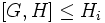# Normality is strongly intersection-closed

This article gives the statement, and possibly proof, of a basic fact in group theory.
View a complete list of basic facts in group theory
VIEW FACTS USING THIS: directly | directly or indirectly, upto two steps | directly or indirectly, upto three steps|
This article gives the statement, and possibly proof, of a subgroup property (i.e., normal subgroup) satisfying a subgroup metaproperty (i.e., strongly intersection-closed subgroup property)
View all subgroup metaproperty satisfactions | View all subgroup metaproperty dissatisfactions |Get help on looking up metaproperty (dis)satisfactions for subgroup properties
Get more facts about normal subgroup |Get facts that use property satisfaction of normal subgroup | Get facts that use property satisfaction of normal subgroup|Get more facts about strongly intersection-closed subgroup property

## Statement

### Verbal statement

An arbitrary (possibly empty) Intersection of subgroups (?) of Normal subgroup (?)s of a group is normal.

Note: The use of the word strongly is to allow the empty intersection as well. We can also say that normality is intersection-closed and also identity-true.

### Symbolic statement

Let$I$ be an indexing set and$H_i$ be a family of normal subgroups of$G$ indexed by$I$. Then, the intersection, over all$i$ in$I$, of the normal subgroups$H_i$, is also a normal subgroup of$G$. In symbols:$\bigcap_{i \in I} H_i \triangleleft G$

## Definitions used

### Normal subgroup

A subgroup$N$ of a group$G$ is said to be normal, if given any inner automorphism$\sigma$ of$G$ (viz a map sending$x$ to$gxg^{-1}$), we have$\sigma(N)$$N$.

### Strongly intersection-closed

A subgroup property is termed strongly intersection-closed if given any family of subgroups having the property, their intersection also has the property. Note that just saying that a subgroup property is intersection-closed simply means that given any nonempty family of subgroups with the property, the intersection also has the property.

Thus, the property of being strongly intersection-closed is the conjunction of the properties of being intersection-closed and identity-true, viz satisfied by the whole group as a subgroup of itself.

## Generalizations

The general result (of which this can be viewed as a special case) is that any invariance property is strongly intersection-closed.

Here, an invariance property is the property of being invariant with respect to a certain collection of functions on the whole group. For normal subgroups, the collection of functions is the inner automorphisms.

## Proof

### Hands-on proof using invariance under inner automorphisms definition

This proof method generalizes to the following results: invariance implies strongly intersection-closed Other particular cases of the generalization include characteristicity is strongly intersection-closed, full invariance is strongly intersection-closed]], and strict characteristicity is strongly intersection-closed.

Given:$H_i$ is a family of normal subgroups of$G$ indexed by$i \in I$.$H = \bigcap_{i \in I} H_i$ is the intersection.

To prove:$H \triangleleft G$. In other words, for any$x$ in$H$ and any inner automorphism$\sigma$ of$G$, we need to show that$\sigma(x) \in H$.

Proof: Since$x$ is in$H$,$x \in H_i \forall i \in I$. By the normality of$H_i$,$\sigma(x) \in H_i \forall i \in I$. Hence,$\sigma(x) \in \bigcap_{i \in I} H_i = H$. This completes the proof.

### Proof using kernel of homomorphism definition

Given:$H_i$ is a family of normal subgroups of$G$ indexed by$i \in I$.$H = \bigcap_{i \in I} H_i$ is the intersection.

To prove:$H$ is normal in$G$, i.e.,$H$ occurs as the kernel of some homomorphism originating from$G$.

Proof: For each$i \in I$,$H_i$ is the kernel of some homomorphism$f:G \to L_i$, where$L_i$ ca nbe taken as the quotient group$G/H_i$.

Let$L$ be the external direct product (unrestricted) of the$L_i$s and define$f:G \to L$ as the unique map such that for any$g \in G$, the$L_i$-coordinate of$f(g)$ equals$f_i(g)$. Since each$f_i$ is a homomorphism,$f$ is also a homomorphism. Further, the kernel of$f$ is the set of those$g \in G$ for which each$f_i(g)$ is the identity element, which is the intersection of the$H_i$s, which is$H$. Thus,$H$ is the kernel of a homomorphism originating from$G$.

### Proof using commutator definition

Other results proved in a very similar way include commutator-in-center is intersection-closed.

Given:$H_i$ is a family of normal subgroups of$G$ indexed by$i \in I$.$H = \bigcap_{i \in I} H_i$ is the intersection.

To prove:$H$ is normal in$G$, i.e.,$[G,H]$ is contained in$H$.

Proof: Since$H \le H_i$ for each$i \in I$,$[G,H] \le [G,H_i]$ for each$i \in I$. Since each$H_i$ is normal in$G$,$[G,H_i] \le H_i$. Thus,$[G,H] \le H_i$ for each$i$, forcing$[G,H] \le \bigcap_{i \in I} H_i = H$. This completes the proof.

## Consequences

A consequence of normality being strongly intersection-closed is the fact that given any subgroup we can talk of the smallest normal subgroup containing that subgroup. This smallest normal subgroup is termed the normal closure.